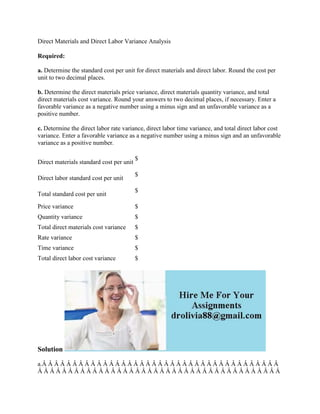Ce diaporama a bien été signalé.
Le téléchargement de votre SlideShare est en cours. ×

# Direct Materials and Direct Labor Variance Analysis Required- a- Deter.docx

Publicité
Publicité
Publicité
Publicité
Publicité
Publicité
Publicité
Publicité
Publicité
Publicité
PublicitéChargement dans…3
×

## Consultez-les par la suite

1 sur 2 Publicité

# Direct Materials and Direct Labor Variance Analysis Required- a- Deter.docx

Direct Materials and Direct Labor Variance Analysis
Required:
a. Determine the standard cost per unit for direct materials and direct labor. Round the cost per unit to two decimal places.
b. Determine the direct materials price variance, direct materials quantity variance, and total direct materials cost variance. Round your answers to two decimal places, if necessary. Enter a favorable variance as a negative number using a minus sign and an unfavorable variance as a positive number.
c. Determine the direct labor rate variance, direct labor time variance, and total direct labor cost variance. Enter a favorable variance as a negative number using a minus sign and an unfavorable variance as a positive number.
Solution
a.Â Â Â Â Â Â Â Â Â Â Â Â Â Â Â Â Â Â Â Â Â Â Â Â Â Â Â Â Â Â Â Â Â Â Â Â Â Â Â Â Â Â Â Â Â Â Â Â Â Â Â Â Â Â Â Â Â Â Â Â Â Â Â Â Â Â Â Â Â Â Â Â Â Â Â Â Â Â Â Â Â Â Â Â Â Â Â Â Â Â Â Â Â Â Â Â Â Â Â Â Â Â Â Â Â Â Â Â Â Â Â Â Â Â Â Â Â Â Â Â Â Â Â Â Â Â Â Â Â Â  Standard
Materials and
Labor Cost
per Faucet
Direct materials (\$22
.

Direct Materials and Direct Labor Variance Analysis
Required:
a. Determine the standard cost per unit for direct materials and direct labor. Round the cost per unit to two decimal places.
b. Determine the direct materials price variance, direct materials quantity variance, and total direct materials cost variance. Round your answers to two decimal places, if necessary. Enter a favorable variance as a negative number using a minus sign and an unfavorable variance as a positive number.
c. Determine the direct labor rate variance, direct labor time variance, and total direct labor cost variance. Enter a favorable variance as a negative number using a minus sign and an unfavorable variance as a positive number.
Solution
a.Â Â Â Â Â Â Â Â Â Â Â Â Â Â Â Â Â Â Â Â Â Â Â Â Â Â Â Â Â Â Â Â Â Â Â Â Â Â Â Â Â Â Â Â Â Â Â Â Â Â Â Â Â Â Â Â Â Â Â Â Â Â Â Â Â Â Â Â Â Â Â Â Â Â Â Â Â Â Â Â Â Â Â Â Â Â Â Â Â Â Â Â Â Â Â Â Â Â Â Â Â Â Â Â Â Â Â Â Â Â Â Â Â Â Â Â Â Â Â Â Â Â Â Â Â Â Â Â Â Â  Standard
Materials and
Labor Cost
per Faucet
Direct materials (\$22
.

Publicité
Publicité

### Direct Materials and Direct Labor Variance Analysis Required- a- Deter.docx

1. 1. Direct Materials and Direct Labor Variance Analysis Required: a. Determine the standard cost per unit for direct materials and direct labor. Round the cost per unit to two decimal places. b. Determine the direct materials price variance, direct materials quantity variance, and total direct materials cost variance. Round your answers to two decimal places, if necessary. Enter a favorable variance as a negative number using a minus sign and an unfavorable variance as a positive number. c. Determine the direct labor rate variance, direct labor time variance, and total direct labor cost variance. Enter a favorable variance as a negative number using a minus sign and an unfavorable variance as a positive number. Direct materials standard cost per unit \$ Direct labor standard cost per unit \$ Total standard cost per unit \$ Price variance \$ Quantity variance \$ Total direct materials cost variance \$ Rate variance \$ Time variance \$ Total direct labor cost variance \$ Solution a.Â Â Â Â Â Â Â Â Â Â Â Â Â Â Â Â Â Â Â Â Â Â Â Â Â Â Â Â Â Â Â Â Â Â Â Â Â Â Â Â Â Â Â Â Â Â Â Â Â Â Â Â Â Â Â Â Â Â Â Â Â Â Â Â Â Â Â Â Â Â Â Â Â Â Â Â Â Â Â
2. 2. Â Â Â Â Â Â Â Â Â Â Â Â Â Â Â Â Â Â Â Â Â Â Â Â Â Â Â Â Â Â Â Â Â Â Â Â Â Â Â Â Â Â Â Â Â Â Â Â Â Â Â Standard Materials and Labor Cost per Faucet Direct materials (\$22